Single Layer Graphene Sheet-based Nanoelectromechanical Resonator as Mass Detection | OMICS International
Journal of Lasers, Optics & Photonics

# Single Layer Graphene Sheet-based Nanoelectromechanical Resonator as Mass Detection

Aziz NM1*, Wael IAS2 and Adel HP3

1Beni-Suef University, Beni-Suef, Egypt

2Faculty of Engineering, Tor Vergata University, Rome, Italy

3Faculty of Engineering, Ain-Shams University, Cairo, Egypt

*Corresponding Author:
Aziz NM
Faculty of Science
Beni-Suef University
Beni- Suef, Egypt
Tel: +011-4799-8837
E-mail: [email protected]

Received Date: September 22, 2015; Accepted Date: November 30, 2015; Published Date: December 05, 2015

Citation: Aziz NM, Wael IAS, Adel HP (2015) Single Layer Graphene Sheet-based Nanoelectromechanical Resonator as Mass Detection. J Laser Opt Photonics 2:125. doi:10.4172/2469-410X.1000125

Copyright: © 2015 Aziz NM, et al. This is an open-access article distributed under the terms of the Creative Commons Attribution License, which permits unrestricted use, distribution, and reproduction in any medium, provided the original author and source are credited.

Visit for more related articles at Journal of Lasers, Optics & Photonics

#### Abstract

Mass detection of molecules using single layer graphene sheet is investigated in the present paper. A nanoelectromechanical system resonator device is proposed which is modeled as single layer graphene coupled to electronic transport through such device via two metallic leads. The conductance of such device is deduced by solving eigenvalue differential equation. The influence of both photon energy of an induced ac-field and magnetic field are taken into consideration. The present results show that both the resonant frequency shift and the quality factor are very sensitive to the mass of certain molecule. Also, the photon energy of the induced ac-field enhances the sensitivity of these parameters. The present research is very important for detecting the mass of both chemical molecules and bio-molecules. This can be achieved experimentally by measuring the quantum conductance of the present device, which is related to the resonant frequency shift and the quality factor.

#### Keywords

Single layer graphene; Nanoelectromechanical systems resonator; Resonant frequency shift; Quality factor; Induced ac-field

#### Introduction

The advances in nanotechnology have brought new tools to the field of electronics and sensors. New designed materials offer new and unique properties enabling the development and cost efficient production of state of the art components that operate faster, has higher sensitivity, consume less power, and can be packed at much higher densities. With their nanometer scale geometry sizes and high operation frequencies, nanoelectromechanical systems (NEMS) hold great promises for much advanced performances in a broad range of applications [1,2]. To date, they have been served in many unprecedented applications such as mass spectrometry [3,4], and nanobiotechnology [5,6]. Mass spectrometry is an analytical technique used for determining the mass of both chemical and biological molecules and the elemental composition of molecules. The mass sensing mechanism of a mechanical resonator is the resonant frequency, which shifts when a mass is loaded. The micro/ nano-mechanical resonator sensors, which can detect the adsorption of a protein , a bio-molecule , a cell , a virus  and an atom , have been developed.

Graphene is a single two-dimensional layer of carbon atoms bound in a hexagonal lattice structure [9,10]. An atom thick graphene sheet comprised of a hexagonal network of covalently bonded carbon atoms is expected to exhibit novel mechanical and electronic properties [11,12]. Graphene has high carrier mobility  which leads to high speed nanoelectronics [10,14,15]. Graphene, a single-atom-thick sheet of graphite that combines aspects of semiconductors and metals, is a promising replacement for conventional semiconductor materials such as silicon. It consists of sp2-hybridized carbon atoms arranged in a two-dimensional (2D) honeycomb crystal structure [9,10]. At present, there are numerous publications devoted to investigation of nanoelectromechanical graphene-based resonators [16-19]. The authors [20,21] investigated the mass sensing of bio-molecules using single walled carbon nanotube-based nanoelectromechanical resonator. The purpose of the present paper is devoted for mass detection of molecules using single layer graphene sheet based nanoelectromechanical resonator.

The model

The nanoelectromechanical system (NEMS) resonator device is modeled as a single layer graphene sheet coupled to electronic transport through such device via two leads. The electron tunneling through such device is studied under the effect of both the magnetic field and an induced ac-field of certain frequency. It is known that this induced acfield with frequency, ω, can induce additional tunneling process when electrons exchange energy by absorbing and /or emitting photons of energy, ħω, where ħ is the reduced Planck’s constant. This kind of tunneling is known as the photon-assisted tunneling (PAT) .

Now, in order to detect the attached molecule and determining its mass, this can be achieved by activating the graphene sheet to vibrate. The resonant frequency shift, Δf, and the quality factor, Q, might be expressed as [20,21,23]: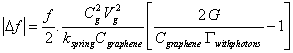(1)

and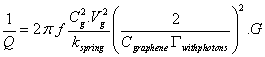(2)

Where Cg is the coupling gate capacitance with graphene sheet, Cgraphene is the graphene capacitance, Vg is the gate voltage, Γwith photons is the electronic tunneling probability, and G is the electronic conductance of graphene. In Equation 1 and 2, the parameter, f, represent the resonant frequency of vibrating graphene sheet and kspring is the spring constant of the vibrating graphene sheet. The conductance (see Equation 1 and 2) of the present device had been calculated using the following Landauer-Buttiker formula [24-26]:(3)

Where δ is the phase of the scattered electrons and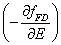is the first derivative of the Fermi-Dirac distribution function and it is given by: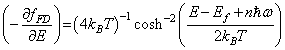(4)

Where kB is the Boltzmann constant and T is the absolute temperature. In Equation 3, the Γwithphotons (E) represents the tunneling probability induced with the photons of ac-field and its expression is given as [25,26]: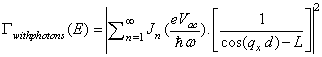(5)

Where Jn is the nth order of Bessel function of first kind, Vac is the amplitude of the external ac-field, and d is the width of the barrier. The parameters qx and L are given as respectively: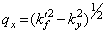(6)

and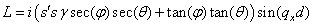(7)

in which γ is expressed as: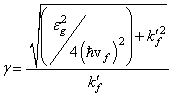(8)

and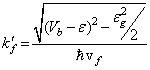(9)

Where εg is the energy gap of graphene and ε is expressed as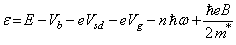(10)

The transport of quasiparticle Dirac fermions are influenced by applying both magnetic field, B, and an ac-field of amplitude, Vac, and of frequency, ω, Vsd is the bias voltage, Vb is the barrier height, and m* is the effective mass of quasiparticle Dirac fermions, E is the scattered energy of quasi- particle Dirac fermions, s = sgn(E) is the signum function of E and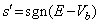and vf is the Fermi velocity. The components of the wave vectors kx and ky outside the barrier are expressed in terms of the angle of incidence,ϕ, of the quasiparticles Dirac fermions as:(11)

Where kf is the Fermi wave vector. In Equation 7 the angle θ is expressed as [25,27]: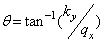(12)

Graphene is the elastic material used for the construction of the resonator [25,26]. The resonant frequency, f, of the vibrating graphene sheet is given as [17,27]: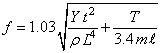(13)

Where Y is Young’s modulus of graphene, ℓ is the length of the graphene sheet, t is its thickness, ρ is its density, m is the mass of the attached molecule, and T is the Tension.

#### Results and Discussion

The present proposed graphene resonator device based mass detection will be analyzed numerically as follows: Numerical calculations are performed for resonant frequency, f. (Equation 13) and the conductance, G,(Equation 3). For monolayer graphene, the values of both barrier height, Vb, and its width are respectively Vb = 120 meV and d = 80 nm [28-30]. Also, the value of the Fermi-velocity, vf is approximately 106 m/s, and the effective mass of quasiparticle Dirac fermions is approximately m* = 0.054 me, where me is the mass of free electron [28-30], Cg ≈ 0.4 nF, Cgraphene ≈ 1.8 fF [10,11], the photon energy, ħω, equals 0.3 eV and B = 2 T. The optimum value of the angle of incidence, ϕ, as indicated by the authors Ahmed et al. [25,26] is, approximately, ϕ ≈ 1 rad(57.3°). The energy gap of single layer graphene, εg, (Equation 9) is computed for different strain values [25,26]. Also, the values of parameters in Equation 13 [12,17-19] are: Y = 1 TPa, ρ = 2200 kg/m3, t = 10 nm, ℓ = 80 μm. The values of both the length and thickness of graphene sheet represent the optimum values for our model in order to give an optimum value of the resonant frequency, f, (Equation 13) [12,17-19].

The features of our results are the following:

Figure 1 shows the variation of the conductance, G, of the present device with the gate voltage, Vg, As shown from this Figure 1 that a periodic oscillation of the conductance with the gate voltage, taking into consideration the effect of both magnetic field, B, and the photon energy of the induced ac-field, ħω, (mid-Infrared radiation). According to the present model of nanoelectronics graphene device, the interaction of the tunneled electrons with the induced ac-field leads to photon-mediated transmission resonances. The interplay between the induced photons and the tunneled electrons leads to what it is known as Fano-resonance [24,31]. In general, the oscillatory behavior of the conductance might be due to Fano-resonance as the quantum transport of electrons is performed from continuum states of leads to the discrete states of nanostructure graphene sheet .

Figure 2 shows the variation of the resonant frequency, f, of the vibrating graphene sheet with the mass of the attached molecule, m, (Equation 13). As shown from this figure that this resonant frequency decreases as the mass of the attached molecule increases. It must be noted that when calculating the resonant frequency, f, (Equation 13), we consider the position of the attaché mass is random on the graphene sheet during vibrating it. This is accomplished by relating the tension, T, (see Equation 13), to the strain, ε0, [32,33] due to the attached mass as:

Figure 2: The variation of the resonant frequency with the mass of the attached molecule.

Τ = ε0 Υ S (14)

Where S is the area of the graphene sheet and ε0 is the strain.

Figure 3 shows the variation of the resonant frequency shift, Δf, (Equation 1) with the mass of the attached molecule. As shown from this figure that the resonant frequencies shift decreases as the mass of the attached molecule increases. These ranges of frequencies are consistent with the photon energy, ħω = 0.3 eV, (mid infrared region) of the induced ac-field. This coupling between the mechanical vibration of the graphene sheet and the influenced photon energy affect prominently on the mass sensitivity in the range of zepto-gram [20,21].

Figure 3: The variation of the resonant frequency shift, ?f, with the mass of the attached molecule.

Figure 4 shows the variation of the inverse of quality factor, (1/Q), with the mass of the attached molecule. As shown from this figure that the inverse quality factor decreases as the mass of the attached molecule increases. The higher quality factor (Q-factor) means higher sensitivity and more reliable performance of the present single layer graphene sheet based nanoelectromechanical systems (NEMS) resonator [3,19]. These results also show the promise of graphene for mass sensing of different types of molecules, for example, chemical gas molecules and bio-molecules.

Figure 4: The variation of the reciprocal of quality factor with the mass of the attached molecule.

#### Conclusion

Because of its unique material properties, graphene is an ideal candidate for a resonator material. It has very high Young’s modulus, is naturally one atom thick, and despite its miniscule thickness, is highly electrically conductive, and nearly impermeable to gasses and liquids. Results in the present paper show that the resonant frequency shift and the quality factor are sensitive to the mass of the attached molecules in the range of zepto-gram. So, single layer graphene sheet based such nanoelectromechanical resonator might be designed to target the detection of the mass of different types of molecules.

#### References

Select your language of interest to view the total content in your interested language

### Article Usage

• Total views: 8614
• [From(publication date):
December-2015 - Dec 14, 2019]
• Breakdown by view type
• HTML page views : 8453Can't read the image? click here to refresh